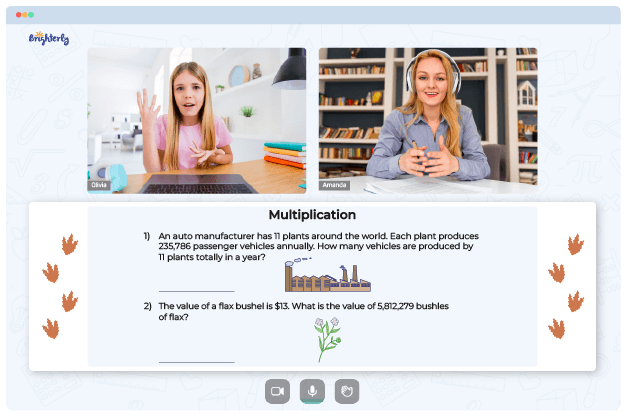# Multiplying Integers Worksheets

The best approach to learn how to multiply integers is by practicing with the multiplying integers worksheets. Multiplying integers worksheets may help graders learn about integers, explore their examples, and understand how to carry out the operation.

## Free multiply integers worksheet

Integers will remain a significant part of the math curriculum throughout a student’s academic career. To help your kids learn and master the concept, you can get free versions of worksheets online from the Brighterly’s website. The worksheet will give your kids a solid grasp of integers and teach how to solve them.

Teachers can use multiplying integers worksheets to raise excitement in their classroom. They can divide the class and make teams compete using the worksheets.

Math for Kids

Is Your Child Struggling With Math?
1:1 Online Math TutoringThough multiplication is usually challenging for kids, the use of worksheets is an easy route to learning the topic. Brighterly’s multiplying integers worksheets comprise questions ranging from simple to complex ones. The questions are arranged so kids learn sequentially. This approach is the best as the questions do not overwhelm students.

Brighterly’s multiplying integers worksheets are designed by leading professionals with sound knowledge of effective learning approaches. Also, the bright design and exciting approach of the sheets ensures your kids will learn while having fun.

## Printable multiplication of integers worksheet### Multiplying Integers Worksheets PDF

Multiplying Integers Worksheet### Multiplying Integers Worksheets PDF

Multiplication Of Integers Worksheet### Multiplying Integers Worksheets PDF

Multiply Integers Worksheet### Multiplying Integers Worksheets PDF

Multiplying Integers Worksheets

You can get the printable version of the multiplying integers worksheets from the Brighterly’s website. There are multiplying integers worksheet grade 7 and lower grade kids use. So, irrespective of your kid’s level, you will find a suitable resource.

With the paper format of the worksheet, your kids will learn to study repeatedly without supervision. Since the sheet comes with answers, they will adjust when they perform poorly. Repeated practice with the multiplying integers worksheets will boost their self-confidence and help them become experts.

### More Integers Worksheets

Need help with Multiplication?• Is your child having trouble understanding multiplication concepts?
• An online tutor could provide the necessary guidance.

Does your child need extra assistance with grasping of multiplication? Start learning with an online tutor.